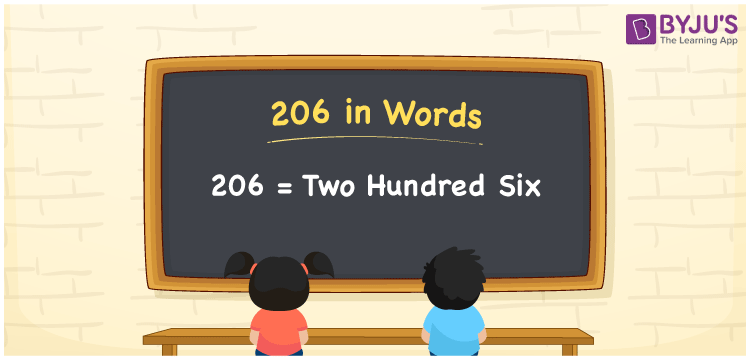# 206 in words

206 in words is written as Two Hundred and Six. 206 represents the count or value. The article on Counting Numbers can give you an idea about count or counting. The number 206 is a 3 digit number that is used in expressions related to money, days, distance, length, weight and so on. Let us consider an example for 206. “I have Two Hundred and Six colour marbles.”

 206 in words Two Hundred and Six Two Hundred and Six in Numbers 206

## 206 in English Words## How to Write 206 in Words?

We can convert 206 to words using a place value chart. The number 206 has 3 digits, so let’s make a chart that shows the place value up to 3 digits.

 Hundreds Tens Ones 2 0 6

Thus, we can write the expanded form as:

2 × Hundred + 0 × Ten + 6 × One

= 2 × 100 + 0 × 10 + 6 × 1

= 206

= Two Hundred and Six.

206 is the natural number that is succeeded by 205 and preceded by 207.

206 in words – Two Hundred and Six.

Is 206 an odd number? – No.

Is 206 an even number? – Yes.

Is 206 a perfect square number? – No.

Is 206 a perfect cube number? – No.

Is 206 a prime number? – No.

Is 206 a composite number? – Yes.

## Solved Example

1. Write the number 206 in expanded form

Solution: 2 × 100 + 0 × 10 + 6 × 1

We can write 206 = 200 + 00 + 6

= 2 × 100 + 0 × 10 + 6 × 1.

## Frequently Asked Questions on 206 in words

Q1

### How to write the number 206 in words?

206 in words is written as Two Hundred and Six.
Q2

### Is 206 divisible by 3?

No. 206 is not divisible by 3.
Q3

### Is 206 a prime number?

No. 206 is not a prime number.